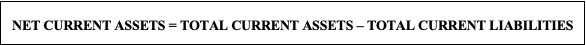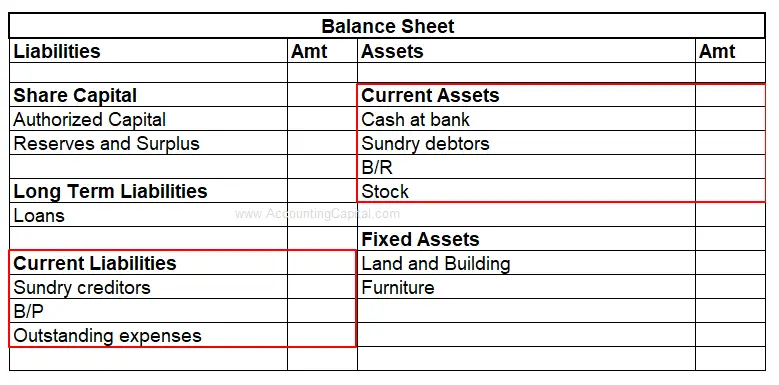# What is the formula to calculate net current assets?

-This question was submitted by a user and answered by a volunteer of our choice.

## Meaning of Net Current Assets

In simple terms, Net Current Assets refer to the total amount of current assets, excluding the total amount of current liabilities in a business. It can also be referred to as Net Working Capital.

The Net Current Assets can have a positive or a negative value, wherein the two are an indicator of the well-being of a business. In case the current assets are greater than the current liabilities, the company possesses sufficient assets to pay off its indebtedness and is operating efficiently.

However, a company is said to be facing financial difficulty and is not in a position to pay off its debts when the value of net current assets is negative.

## Calculation of Net Current Assets: Formula

The formula is as follows:where;

Total current assets = Cash and Cash Equivalents + Stock + Marketable Securities +                                          Prepaid Expenses + Accounts Receivable + Other Liquid Assets

Total current liabilities = Current Portion of Long-term Debt + Notes Payable +                                                      Accounts Payable + Accrued Expenses + Unearned Revenue +                                          Other Short-term Debt

• On the balance sheet, the total current assets are listed under the heading current assets.
• On the other hand, the total current liabilities are shown on the balance sheet under the heading current liabilities.

On the balance sheet, components of net current assets i.e., current assets and current liabilities appear on the asset side and liability side respectively.

Current assets are directly proportional to net current assets whereas current liabilities are inversely proportional to net current assets.### Example

Calculate the Net Current Assets of ABC Ltd.

(Extract of Balance Sheet)

 PARTICULARS AMOUNT CURRENT ASSETS Cash and Cash Equivalents 2,00,000 Accounts Receivables 40,000 Stock Inventory 15,000 Marketable Securities 35,000 Prepaid Expenses 6,000 TOTAL CURRENT ASSETS 2,96,000 CURRENT LIABILITIES Accounts Payable 15,000 Accrued Expense 2,000 Unearned Revenue 20,000 Taxes Payable 40,000 Short-term Debt 10,000 Interest Payable 6,000 TOTAL CURRENT LIABILITIES 93,000

Solution:

Total current assets = 2,96,000

Total current liabilities= 93,000

Net Current Assets = Total Current Assets – Total Current Liabilities

= 2,96,000- 93,000

= 2,03,000

Key Takeaways

• Net Current Assets are also known as Net Working Capital.
• Net Current Assets is the difference between the total current assets and total current liabilities.

## Net Current Assets Vs Current Assets

 Basis Net Current Assets Current Assets Meaning It is the difference between total current assets and total current liabilities As the name implies, current assets refer to short-term assets that will be used, sold or converted to cash within one year by a company. Formula Current Assets – Current Liabilities Cash and Cash Equivalents + Stock + Marketable Securities + Prepaid Expenses + Accounts Receivable + Other Liquid Assets Other Name It is also known as working capital. It is also known as liquid assets.

###### Subscribe
* indicates required